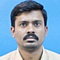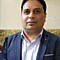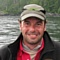Project Management Central

Project status based on schedule, work completeNetwork:0Hello, I am hoping to get some ideas from the group. I was wondering what are the standards for calculating project status based on schedule only? I am not tracking budget or costs. This is for a simple project. Someone recommended: Expected progress / % Complete (or some variation of it). For example, is a project is 80% complete but should be 90%, we would compute 90/80 = 1.125. Status can then be determined as Off Track where: On Track = 0 - .7; At Risk = 7. – 1; Off Track 1.

Thank you!!
Sort By:
Page: 1 2 3 next>Network:7256Using EVM gives a correct assessment of Project Status.Network:0Hi Rajesh,

We are not tracking budget or cots at this stage. I am trying to calculate status based on schedule only.

Thank you,
JuliaNetwork:2871Earned Value Method is very useful to calculate the project status in term of schedule variance and cost variance . Try it.Network:0We are not keeping track of the budget/costs at this stage. As far as I know EVM is based on cost, correct? How can I calculate project status on schedule only?

Thank you,
Julia
...
1 reply by Rami Kaibni
Jul 25, 2019 2:35 AM
Rami Kaibni
...Network:337Julia,
EVM doesn't have to be measured in cost. It is comparing what you planned to get done in a period of time, to what you got done in that time. You can apply cost to the effort, but it really is progress to plan, rather than cost vs. time.Network:0Hi Keith,

Thank you for your reply. Can you please send me a formula and/or some parameters? I'm a bit lost.

Thank you,
JuliaNetwork:126080Jul 25, 2019 1:17 AM
Replying to Julia Braga
...

We are not keeping track of the budget/costs at this stage. As far as I know EVM is based on cost, correct? How can I calculate project status on schedule only?

Thank you,
JuliaNetwork:126080Julia, EVM can measure Schedule Variance (SV) and Schedule Performance Index (SPI). It is not only for cost measurement:

SV = EV - PV
SPI = EV / PV

Those variables has nothing to do with cost spent but mainly has to do with your budget.

For example: As per your plan for this cut off date, you are scheduled you finish X amount of scope that is equivalent to \$Y in budget. However, you finished Z amount of scope that is equivalent to \$W amount in budget so you can calculate the following:

SV = \$W - \$Z (If negative then you are behind schedule)
SPI = \$W/\$Z (If less than one then you are behind schedule, 0 you are on schedule, positive then ahead)

You basically did not track costs at all but you just measured the scope completed vs the scope planned in terms of budget.

If you don’t want to use budget and depending on your project then for example: As per schedule, You are supposed to finish painting 100 sq.ft in July 2019, however, you finished only 90 sq.ft so you do calculate either SV or SPI and you will figure out your progress and will be able then to calculate your Estimate to a Complete (ETC).

The only thing that EVM doesn’t measure is if you are delivering value so being ahead of schedule and be;ow budget as per EVM calculations doesn’t mean you are delivering value.

Hope this helps.Network:161There are a lot of calculative formulas for EVM, just like given in below:
CP = EV - AC
CPI = EV/AC
SV = EV - PV
SPI = EV/PV
EAC =
1) EAC = AC + Bottom-up ETC
2) EAC =BAC/Cumulative CPI
3) EAC = AC + (BAC - EV)
4) EAC = AC + [BAC - EV / (Cumulative CPI x Cumulative SPI)]
TCPI = (BAC – EV) / (BAC – AC).
VAC = BAC - EAC

For Schedule:
Straight Average = ( P + M+ O) / 3
Beta Average = ( P + 4M+ O) / 6

Communication Channel = n * (n-1) / 2
...
1 reply by Julia Braga
Jul 25, 2019 6:31 PM
Julia Braga
...
Thank you.Network:0HI Rami,

Thank you for your reply but I still don't understand how I will calculate SV or SPI without a budget. I am assuming I have enough money to do everything. It's just a scheduling issue.

Thank you,
Julia
...
1 reply by Rami Kaibni
Jul 25, 2019 3:18 AM
Rami Kaibni
...
Julia, in this case you need to define another metric to measure the Schedule such as Square Foot as in the second example I gave you, or Unit Code in Software or Cubic Meter in Concrete and so on but without defining a certain metric to measure your Schedule progress, you will never be able to measure it using EVM.

Regarding your comment, even if you assume you have enough money, what I just explained to you above has to do with how much money you allocated or budgeted, not if there is enough money or not so if you are working on a project without a budget then you certainly need to define an alternative meaningful metric to measure progress / schedule but make sure that this metric is consistent throughout all your project, Hope this clarifies !
Page: 1 2 3 next>